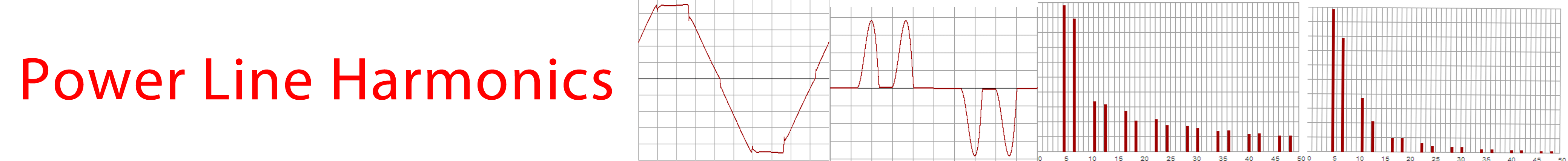# Power Line Harmonics.

## What is a Harmonic?

A harmonic is a signal that is at a frequency that is a direct whole number multiple of the original frequency and occuring at the same time as the original frequency.
Any repetitive non sinusoidal waveform can be shown to be made up of a combination of a fundamental sinusoidal waveform and harmonic sinusoidal waveforms.
Harmonics are given a harmonic number which is the multiple of the fundamental frequency. On a 50Hz supply, the third harmonic is at 150Hz and the second at 100Hz.
Harmonics are described as even harmonics and add harmonics depending on their harmonic number. Symmetric distortion contributes odd order harmonics and assymetric distortion contributes even harmonics.

## What are voltage harmonics?

Distorted voltage waveforms contain voltage harmonics.

## What are current harmonics?

Non sinusoidal current waveforms are made up of a fundamental sinewave current plus harmonic currents.

## What do Harmonics do?

Harmonics on the electrical supply system cause increased losses and can excite resonance in some circuits resulting in elevated voltages.
Harmonic voltages will cause harmonic currents to flow in resistive loads that are proportional to the harmonic voltage, but in capacitive loads, the harmpnic currents will increase as the frequency of the harmonic increases. This is because the impedance of a capacitor is inversly proportional to the applied frequency. The increased current in capacitors wil increase the capacitor heating and can cause premature failure
Harmonic voltages cause an increase in the losses in iron cored components and can result in a major increase in the rotor losses of an induction motor.
Supply distribution transformers must be derated in the presence of harmonic currents in order to avoid transformer over heating.
Harmonic currents cause a distortion power factor less than 1.0 and this affects the supply in much the same way as a displacement power factor.

## What causes harmonics?

Harmonic currents are cuased by non linear loads and appliances. Commonly, the non linear components are solid state rectifiers used in all manner of modern equipment.
The most common source of major harmonic currents are the rectifier circuits in variable frequency drives, and the rectifier circuits in switchmode power supplies as are used in many industrial supplies and most modern domestic appliances and computers.
Harmonic voltages are generated by the action of harmonic currents on the impedance of the electrical supply.